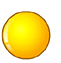# php引用(&)详解及注意事项

## 摘自：PHP引用(&)使用详解

php的引用（就是在变量或者函数、对象等前面加上&符号）

1.变量的引用

PHP 的引用允许你用两个变量来指向同一个内容

<?

$a="ABC";$b =&$a; echo$a;//这里输出:ABC
echo $b;//这里输出:ABC$b="EFG";

echo $a;//这里$a的值变为EFG 所以输出EFG
echo $b;//这里输出EFG ?> 2.函数的引用传递（传址调用 传址调用我就不多说了 下面直接给出代码 <?php function test(&$a)
{

$a=$a+100;
}

$b=1; echo$b;//输出１
test($b); //这里$b传递给函数的其实是$b的变量内容所处的内存地址，通过在函数里改变$a的值　就可以改变$b的值了 echo "<br>"; echo$b;//输出101
?>

上面的“ test($b); ” 中的$b前面不要加 & 符号，但是在函数“call_user_func_array”中，若要引用传参，就得需要 & 符号，如下代码所示：

<?php

function a(&$b){$b++;
}
$c=0; call_user_func_array('a',array(&$c));

echo $c; //输出 1 ?> 3.函数的引用返回 先看代码 <?php function &test() { static$b=0;//申明一个静态变量
$b=$b+1;

echo $b; return$b;
}

$a=test();//这条语句会输出$b的值　为１
$a=5;$a=test();//这条语句会输出　$b的值 为2$a=&test();//这条语句会输出　$b的值 为3$a=5;
$a=test();//这条语句会输出$b的值　为6
?>

$a=test()方式调用函数，只是将函数的值赋给$a而已，　而$a做任何改变 都不会影响到函数中的$b

$a=&test();$a=5;

public function & get(){

return $this->data; } public function out(){ echo$this->data;
}

}

$aa = new talker();$d = &$aa->get();$aa->out();
$d = 'How';$aa->out();
$d = 'Are';$aa->out();
$d = 'You';$aa->out();
?>

the output is
"HiHowAreYou"

4.对象的引用

<?php

class a{

var $abc="ABC"; }$b=new a;

$c=$b;

echo $b->abc;//这里输出ABC echo$c->abc;//这里输出ABC
$b->abc="DEF"; echo$c->abc;//这里输出DEF
?>

PHP5中默认就是通过引用来调用对象， 但有时你可能想建立一个对象的副本，并希望原来的对象的改变不影响到副本 . 为了这样的目的，PHP5定义了一个特殊的方法，称为__clone

function __construct($str){$this->name = $str; } function __toString(){ return 'my name is "'.$this->name .'" and I live in "' . __CLASS__ . '".' . "\n";
}

function setName($str){$this->name = $str; } } class MasterOne{ protected$foo;

function __construct($f){$this->foo = $f; } function __toString(){ return 'Master: ' . __CLASS__ . ' | foo: ' .$this->foo . "\n";
}

function setFooName($str){$this->foo->setName( $str ); } } class MasterTwo{ protected$foo;

function __construct($f){$this->foo = $f; } function __toString(){ return 'Master: ' . __CLASS__ . ' | foo: ' .$this->foo . "\n";
}

function setFooName($str){$this->foo->setName( $str ); } }$bar = new foo('bar');

print("\n");
print("Only Created \$bar and printing \$bar\n");
print$bar ); print("\n"); print("Now \$baz is referenced to \$bar and printing \$bar and \$baz\n");$baz =& $bar; print$bar );

print("\n");
print("Now Creating MasterOne and Two and passing \$bar to both constructors\n");$m1 = new MasterOne( $bar );$m2 = new MasterTwo( $bar ); print$m1 );
print$m2 ); print("\n"); print("Now changing value of \$bar and printing \$bar and \$baz\n");
$bar->setName('baz'); print$bar );
print$baz ); print("\n"); print("Now printing again MasterOne and Two\n"); print$m1 );
print$m2 ); print("\n"); print("Now changing MasterTwo's foo name and printing again MasterOne and Two\n");$m2->setFooName( 'MasterTwo\'s Foo' );
print$m1 ); print$m2 );

print("Also printing \$bar and \$baz\n");
print$bar ); print$baz );
?>

Only Created $bar and printing$bar
my name is
"bar" and I live in "foo".

Now
$baz is referenced to$bar and printing $bar and$baz
my name is
"bar" and I live in "foo".

Now Creating MasterOne and Two and passing
$bar to both constructors Master : MasterOne | foo: my name is "bar" and I live in "foo". Master : MasterTwo | foo: my name is "bar" and I live in "foo". Now changing value of$bar and printing $bar and$baz
my name is
"baz" and I live in "foo".
my name is
"baz" and I live in "foo".

Now printing again MasterOne and Two
Master
: MasterOne | foo: my name is "baz" and I live in "foo".

Master
: MasterTwo | foo: my name is "baz" and I live in "foo".

Now changing MasterTwo
's foo name and printing again MasterOne and Two
Master: MasterOne | foo: my name is "MasterTwo
's Foo" and I live in "foo".

Master: MasterTwo | foo: my name is
"MasterTwo's Foo" and I live in "foo".

Also printing $bar and$baz
my name is "MasterTwo
's Foo" and I live in "foo".
my name is
"MasterTwo's Foo" and I live in "foo".

$bar = new foo('bar');$m1 = new MasterOne( $bar );$m2 = new MasterTwo( $bar ); 实例对象$m1与$m2中的$bar是对实例$bar的引用，而非拷贝，这是php5中，对象引用的特点，也就是说 1.$m1或$m2内部，任何对$bar的操作都会影响外部对象实例$bar的相关值。 2.外部对象实例$bar的改变也会影响$m1和$m2内部的$bar的引用相关值。 在php4中，要实现如上述的 用一个对象实例去当着另外一个对象的属性时，其等价代码（即引用调用）类似如下： class foo{ var$bar;

function setBar(&$newBar){$this->bar =& newBar;
}
}

5.引用的作用
如果程序比较大,引用同一个对象的变量比较多,并且希望用完该对象后手工清除它,个人建议用 "&" 方式,然后用$var=null的方式清除. 其它时候还是用php5的默认方式吧. 另外, php5中对于大数组的传递,建议用 "&" 方式, 毕竟节省内存空间使用。 6.取消引用 当你 unset 一个引用，只是断开了变量名和变量内容之间的绑定。这并不意味着变量内容被销毁了。例如： <?php$a = 1;

$b =&$a;

unset ($a); ?> 不会 unset$b，只是 $a。 7.global 引用 当用 global$var 声明一个变量时实际上建立了一个到全局变量的引用。也就是说和这样做是相同的：

<?php

$var =&$GLOBALS["var"];
?>

Example  在函数内引用全局变量

<?php
$var1 = "Example variable";$var2 = "";

function global_references($use_globals) { global$var1, $var2; if (!$use_globals) {

$var2 =&$var1// visible only inside the function
} else {

$GLOBALS["var2"=&$var1// visible also in global context
}
}

global_references(
false);
echo "var2 is set to '$var2'\n"// var2 is set to '' global_references(true); echo "var2 is set to '$var2'\n"// var2 is set to 'Example variable'
?>
global $var; 当成是$var =& $GLOBALS['var']; 的简写。从而将其它引用赋给$var 只改变了本地变量的引用。

8.$this 在一个对象的方法中，$this 永远是调用它的对象的引用。

//下面再来个小插曲
php中对于地址的指向（类似指针）功能不是由用户自己来实现的，是由Zend核心实现的，php中引用采用的是“写时拷贝”的原理，就是除非发生写操作，指向同一个地址的变量或者对象是不会被拷贝的。

1:如果有下面的代码

<?

$a="ABC";$b=&$a; ?> 其实此时$a与$b都是指向同一内存地址 而并不是$a与$b占用不同的内存 ２:如果在上面的代码基础上再加上如下代码 <?php$a="EFG";
?>

php的引用（就是在变量或者函数、对象等前面加上&符号）是个高级话题，新手多注意，正确的理解php的引用很重要，对性能有较大影响，而且理解错误可能导致程序错误！

$a = array('a','c'...'n');$b = $a; 如 果程序仅执行到这里，$a和$b是相同的，但是并没有像C那样，$a和$b占用不同的内存空间，而是指向了同一块内存，这就是php和c的差别，并不需要 写成$b=&$a才表示$b指向$a的内存，zend就已经帮你实现了引用，并且zend会非常智能的帮你去判断什么时候该这样处理，什么时候不 该这样处理。 如果在后面继续写如下代码，增加一个函数，通过引用的方式传递参数，并打印输出数组大小。 function printArray(&$arr//引用传递
{

print(count($arr)); } printArray($a);

function printArray($arr) //值传递 { print(count($arr));
}
printArray(
$a); 上面的代码直接传递$a值到printArray()中，此时并不存在引用传递，所以没有出现写时拷贝。## 引用做什么

PHP 的引用允许用两个变量来指向同一个内容。意思是，当这样做时：

<?php $a =&$b; ?> 

Note:

$a$b 在这里是完全相同的，这并不是 $a 指向了$b 或者相反，而是 $a$b 指向了同一个地方。

Note:

Note:

Example #1 对未定义的变量使用引用

<?php function foo(&$var) { } foo($a); // $a is "created" and assigned to null$b = array(); foo($b['b']); var_dump(array_key_exists('b',$b)); // bool(true) $c = new StdClass; foo($c->d); var_dump(property_exists($c, 'd')); // bool(true) ?>  同样的语法可以用在函数中，它返回引用，以及用在 new 运算符中（PHP 4.0.4 以及以后版本）： <?php$bar =& new fooclass(); $foo =& find_var($bar); ?> 

Note:

Warning

<?php function foo(&$var) {$var++; } $a=5; foo($a); ?> 

echo "\nbefore:\n";
echo "\$arr1 == {$arr1}\n";
$arr2 =$arr1;
$arr2++; echo "\nafter:\n"; echo "\$arr1 == {$arr1}\n"; echo "\$arr2 == {$arr2}\n"; 输出： before:$arr1 == 1

after:
$arr1 == 1$arr2 == 2

// Example two

$arr3=array(1);$a=&$arr3; echo"\nbefore:\n"; echo"\$a == $a\n"; echo"\$arr3 == {$arr3}\n";$arr4=$arr3;$arr4++;
echo"\nafter:\n";
echo"\$a ==$a\n";
echo"\$arr3 == {$arr3}\n";
echo"\$arr4 == {$arr4}\n";

before:
$a == 1$arr3 == 1

after:
$a == 2$arr3 == 2
$arr4 == 2 ?> 分析说明： 对于“Example two”，刚开始还以为是个bug，其实仔细推敲，非也，分析如下， 在赋值（拷贝）$arr4=$arr3; 之前，还有个对$arr3的第一个元素建立引用的过程，即

$a=&$arr3;

$a、$arr3、\$arr4 三者其实是引用关系，指向同一个地方。

posted on 2011-09-10 11:59  小炒花生米  阅读(70402)  评论(11编辑  收藏  举报